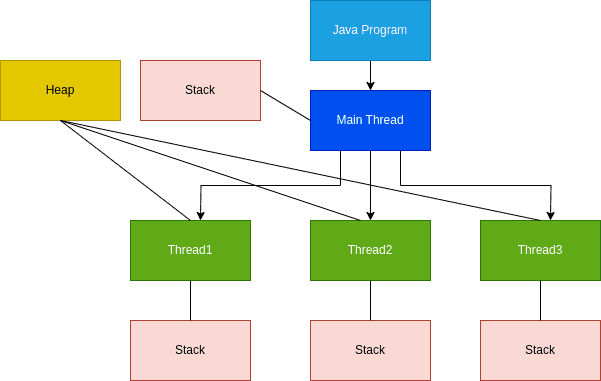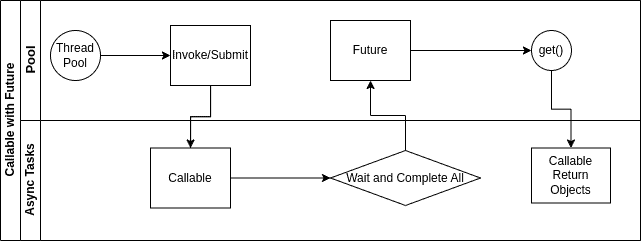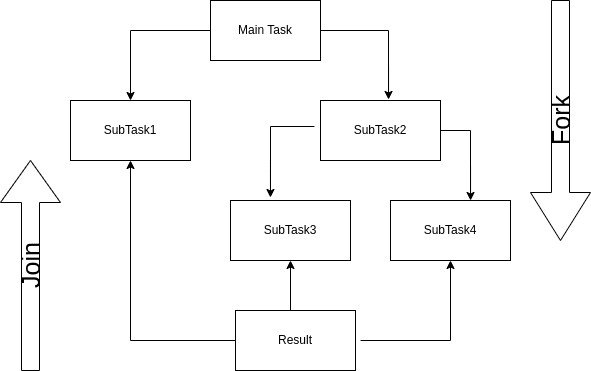### Get started with Spring 5 and Spring Boot 2, through the Learn Spring course:

>> CHECK OUT THE COURSE

## 1. Overview

One of the main features of Java is Concurrency. It allows multiple threads to run and execute parallel tasks. Therefore, we can execute async and non-blocking instructions. This will optimize the available resources, particularly when a computer has multiple CPUs. There are two types of threads: with a return value or without (in the latter case, we say it will have a void return method).

In this article, we’ll focus on how to return a value from a thread that has its job terminated.

We refer to a Java thread as a lightweight process. Let’s have a look at how a Java program usually works:A Java program is a process in execution. A thread is a subset of a Java process and can access the main memory. It can communicate with other threads of the same process.

A thread has a lifecycle and different states. A common way of implementing it is by the Runnable interface:

``````public class RunnableExample implements Runnable {
...
@Override
public void run() {
// do something
}
}``````

Then, we can start our thread:

`````` Thread thread = new Thread(new RunnableExample());

As we can see, we can’t return a value from a Runnable. However, we can synchronize with other threads using wait() and notify(). The join() method will hold the execution in a wait state until it completes. We’ll see later how this is important when we get results from the async execution.

## 3. Callable

Java introduces the Callable interface from version 1.5. Let’s see an example of an async task returning a value of factorial calculation. We are using a BigInteger as the result can be a large number:

``````public class CallableFactorialTask implements Callable<BigInteger> {
// fields and constructor
@Override
public BigInteger call() throws Exception {
return factorial(BigInteger.valueOf(value));
}
}``````

Let’s also create a simple factorial calculator:

``````public class FactorialCalculator {

public static BigInteger factorial(BigInteger end) {
BigInteger start = BigInteger.ONE;
BigInteger res = BigInteger.ONE;

for (int i = start.add(BigInteger.ONE).intValue(); i <= end.intValue(); i++) {
res = res.multiply(BigInteger.valueOf(i));
}

return res;
}

public static BigInteger factorial(BigInteger start, BigInteger end) {
BigInteger res = start;

for (int i = start.add(BigInteger.ONE).intValue(); i <= end.intValue(); i++) {
res = res.multiply(BigInteger.valueOf(i));
}

return res;
}
}``````

A Callable has only one method call() we need to override. That method will return the object of our async task.

Callable and Runnable are both @FunctionalInterface. Callable can return a value and throw exceptions. However, it needs a Future to complete the task.

## 4. Execute Callable

We can execute a Callable using Future or a Fork/Join.

### 4.1. Callable with Future

Since version 1.5, Java has the Future interface to create objects containing the response of our asynchronous processing. We can logically compare a Future to a Promise in Javascript.

For instance, we commonly see Future when we want to get data from multiple endpoints. Therefore, we’ll need to wait for all our tasks to complete to collect the response data.

A Future wraps the response and waits until the threads are completed. However, we might have an interruption, for example, for a timeout or an execution exception.

Let’s look at the Future interface:

``````public interface Future<V> {
boolean cancel(boolean var1);
boolean isCancelled();
boolean isDone();
V get() throws InterruptedException, ExecutionException;
V get(long var1, TimeUnit var3) throws InterruptedException, ExecutionException, TimeoutException;
}``````

The get method is interesting for us to wait and get the result of the execution.

To start a Future job, we associate its execution with a ThreadPool. This way, we’ll allocate some resources for those asynchronous tasks.

Let’s create an example with an Executor that does a sum of factorial numbers from a Callable we have seen earlier. We’ll use an Executor interface and ExecutorService implementation to create a ThreadPoolExecutor. We might want to use a fixed or a cached thread pool. In this case, we’ll go for a cached thread pool to demonstrate:

``````public BigInteger execute(List<CallableFactorialTask> tasks) {

BigInteger result = BigInteger.ZERO;

List<Future<BigInteger>> futures;

try {
} catch (InterruptedException e) {
// exception handling example
throw new RuntimeException(e);
}

for (Future<BigInteger> future : futures) {
try {
} catch (InterruptedException | ExecutionException e) {
// exception handling example
throw new RuntimeException(e);
}
}

return result;
}``````

We can represent this execution with a diagram where we can observe how the thread pool and the Callable interact:The Executor will invoke and collect all in a Future object. Then, we can get one or more results from our async processing.

Let’s test by summing up the result of two factorial numbers:

``````@Test
void givenCallableExecutor_whenExecuteFactorial_thenResultOk() {
assertEquals(BigInteger.valueOf(126), result);
}``````

### 4.2. Callable with Fork/Join

We also have the option to use ForkJoinPool. It still works similarly to the ExecutorSerivce as it extends the AbstractExecutorService class. However, it has a different way to create and organize threads. It forks a task into smaller tasks and optimizes resources so that they’re never idle. We can represent the subtasks with a diagram:We can see the main task will fork into SubTask1, SubTask3, and SubTask4 as the smallest executables. Finally, they’ll join in the final result.

Let’s transform the previous example into one using the ForkJoinPool. We can wrap all in an executor method:

``````public BigInteger execute(List<Callable<BigInteger>> forkFactorials) {
List<Future<BigInteger>> futures = forkJoinPool.invokeAll(forkFactorials);

BigInteger result = BigInteger.ZERO;

for (Future<BigInteger> future : futures) {
try {
} catch (InterruptedException | ExecutionException e) {
// exception handling example
throw new RuntimeException(e);
}
}

return result;
}``````

In this case, we only need to create a different pool to get our futures. Let’s test this with a list of factorial Callable:

``````@Test
void givenForkExecutor_whenExecuteCallable_thenResultOk() {
assertEquals(BigInteger.valueOf(126),
}``````

However, we might also decide how to fork our tasks. We might want to fork our calculation based on some criteria, for example, on an input parameter or a service load.

``````public class ForkFactorialTask extends RecursiveTask<BigInteger> {
// fields and constructor

@Override
protected BigInteger compute() {

BigInteger factorial = BigInteger.ONE;

if (end - start > threshold) {

int middle = (end + start) / 2;

.join()
.multiply(new ForkFactorialTask(middle + 1, end, threshold).fork()
.join()));
}

return factorial.multiply(factorial(BigInteger.valueOf(start), BigInteger.valueOf(end)));
}
}``````

If some threshold applies, we’ll subdivide our main task. We can then use the invoke() method to get the result:

``````ForkJoinPool forkJoinPool = ForkJoinPool.commonPool();

Also, submit() or execute() are an option. However, we always need the join() command to complete the execution.

Let’s also create a test where we subtask our factorial execution:

``````@Test
}``````

In this case, we’ll divide the factorial of 10 into two tasks. The first will calculate from 1 to 5, while the second will go from 6 to 10.

## 5. Completable Future

Since version 1.8, Java has improved multithreading with the introduction of CompletableFuture. It removes boilerplate code from the Future execution and adds features like chaining or combining async results. However, most importantly, we can now do the async computation for any method, so we are not bound to a Callable. Furthermore, we can join together multiple Futures that are semantically different.

### 5.1. supplyAsync()

Using a CompletableFuture can be as simple as:

``````CompletableFuture<BigInteger> future = CompletableFuture.supplyAsync(() -> factorial(BigInteger.valueOf(10)));
...
BigInteger result = future.get();
``````

We don’t need a Callable anymore. We can pass any lambda expression as an argument. Let’s test the factorial method with supplyAsync():

``````@Test
void givenCompletableFuture_whenSupplyAsyncFactorial_thenResultOk() throws ExecutionException, InterruptedException {
CompletableFuture<BigInteger> completableFuture = CompletableFuture.supplyAsync(() -> factorial(BigInteger.valueOf(10)));
assertEquals(BigInteger.valueOf(3628800), completableFuture.get());
}``````

Notice that we’re not specifying any thread pool. In this case, a default ForkJoinPool will be in use. However, we can specify an Executor, for example, with a fixed thread pool:

``````CompletableFuture<String> future = CompletableFuture.supplyAsync(() -> factorial(BigInteger.valueOf(10)), Executors.newFixedThreadPool(1));
``````

### 5.2. thenCompose()

We can also create a chain of sequential Futures. Suppose we have two factorial tasks, and the second needs input from the first one:

``````CompletableFuture<BigInteger> completableFuture = CompletableFuture.supplyAsync(() -> factorial(BigInteger.valueOf(3)))

BigInteger result = completableFuture.get();``````

We can use thenCompose() method to use a return value from a CompletableFuture in the next one of the chain.

Let’s combine the execution of two factorials. For example, we start from 3, giving us a factorial of 6 as the input for the next factorial:

``````@Test
void givenCompletableFuture_whenComposeTasks_thenResultOk() throws ExecutionException, InterruptedException {
CompletableFuture<BigInteger> completableFuture = CompletableFuture.supplyAsync(() -> factorial(BigInteger.valueOf(3)))
assertEquals(BigInteger.valueOf(720), completableFuture.get());
}``````

### 5.3. allOf()

Interestingly, we can execute multiple Futures in parallel using the static method allOf() that accepts an input var-arg.

Collecting async results from multiple executions will be as simple as adding to allOf() and join() to complete the tasks:

``````BigInteger result = allOf(asyncTask1, asyncTask2)

Note that allOf() has a void return type. Therefore, we need to get the results from the single Futures manually. Also, we can run Futures with different return types in the same execution.

To test, let’s join two different factorial tasks. To demonstrate, one has a number input, while the second has a string:

``````@Test
CompletableFuture<BigInteger> asyncTask1 = CompletableFuture.supplyAsync(() -> BigInteger.valueOf(5));
CompletableFuture<String> asyncTask2 = CompletableFuture.supplyAsync(() -> "3");

.join();

assertEquals(BigInteger.valueOf(126), result);
}``````

## 6. Conclusion

In this tutorial, we’ve seen how to return an object from a thread. We saw how to use Callable combined with Future and a pool of threads. A Future wraps the results and waits until all tasks are completed. We have also seen an example of ForkJoinPool to optimize our execution into multiple subtasks.

CompletableFuture from Java 8 works similarly but also offers new features like the possibility to execute any lambda expression. It also allows us to chain and combine the results of our async tasks.

Finally, we’ve tested a simple factorial task with Future, Fork, and CompletableFuture.

As always, we can find working code examples over on GitHub.

### Get started with Spring 5 and Spring Boot 2, through the Learn Spring course:

>> CHECK OUT THE COURSE